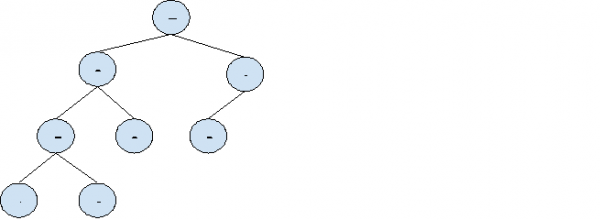# Find if there is a pair in root to a leaf path with sum equals to root's data in C++

In this problem, we are given a Binary Tree. And we need to find if there is a pair in root to a leaf path with sum equals to root’s data.

We need to check if there exists a pair of nodes that lies between root node to leaf nodes such that the sum of values of pairs is equal to the root node’s value.

Let’s take an example to understand the problem,

Input:Output: Yes

Explanation:

Root node value 7

Pairs with sum value equal to root node, (2, 5), (1, 6).

Solution Approach:

We need to traverse the tree and find the pairs using hashing.

For this we will create a hashTable and traverse tree. Insert data into hashtable and then check if its sum with other elements is equal to root.

And at the end, if we find no pairs, return false.

If pairs are found, return true.

## Example

Live Demo

#include<bits/stdc++.h>
using namespace std;

struct Node {

int data;
struct Node* left, *right;
};

struct Node* newnode(int data) {

struct Node* node = new Node;
node->data = data;
node->left = node->right = NULL;
return (node);
}

bool findSumUntill(Node *node, unordered_set<int> &amp;hashTable, int rootVal)
{
if (node == NULL)
return false;

int otherVal = rootVal - node->data;
if (hashTable.find(otherVal) != hashTable.end())
return true;

hashTable.insert(node->data);
bool isFound = findSumUntill(node->left, hashTable, rootVal) || findSumUntill(node->right, hashTable, rootVal);
hashTable.erase(node->data);

return isFound;
}

bool findPairSum(Node *root) {

unordered_set<int> hashTable;

return findSumUntill(root->left, hashTable, root->data) || findSumUntill(root->right, hashTable, root->data);
}

int main()
{
struct Node *root = newnode(7);
root->left = newnode(2);
root->right = newnode(3);
root->left->left = newnode(5);
root->left->right = newnode(9);
root->left->left->left = newnode(1);
root->left->left->right = newnode(6);
root->right->left = newnode(8);

if(findPairSum(root))
cout<<"Pair with sum equal to root value found";
else
cout<<"No pairs found";
return 0;
}

## Output

Pair with sum equal to root value found